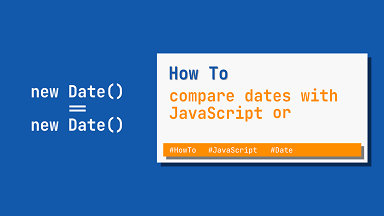# How to Compare Two Dates Using JavaScript

JavaScript allows you to create, modify and work with dates. Sometimes you may need to compare two dates using JavaScript. You can use Date object present in JavaScript and supported by most browsers. In this article, we will learn how to compare two dates using JavaScript.

## How to Compare Two Dates Using JavaScript

The key to comparing dates in JavaScript is to first construct date objects for each date that you want to compare. Here is an example.

```var d1 = new Date();
var d2 = new Date(d1);```

You can also use Date() constructor to create date from strings.

```var d1 = new Date('2022-05-22');
var d2 = new Date("March 21, 2022");```

Once you have created date objects, you can compare it using comparison operators such as ==, !=, ===, and !==.

But for this purpose, you need to call getTime() function on your Date object. Here are a couple of examples to check if our dates d1 and d2 are same or different.

```var same = d1.getTime() === d2.getTime();
var notSame = d1.getTime() !== d2.getTime();```

Please note, you cannot use comparison operators directly on date objects, it may give you wrong output.

```var d1 = new Date();
var d2 = new Date(d1);

console.log(d1 == d2);   // prints false (wrong!)
console.log(d1 === d2);  // prints false (wrong!)
console.log(d1 != d2);   // prints true  (wrong!)
console.log(d1 !== d2);  // prints true  (wrong!)
console.log(d1.getTime() === d2.getTime()); // prints true (correct)```

In this article, we have learnt how to compare two dates using JavaScript’s comparison operators. The key is to first create a Date object for your date and then use comparison operator on it using getTime() function.